# Kraken Daily Market Report for April 20 2021

## Overview

• Total spot trading volume at $3.57 billion, 91% above the 30-day average of$1.87 billion.
• Total futures notional at $927.9 million. • The top five traded coins were, respectively, Bitcoin, Dogecoin, Ethereum, Tether, and Ripple. • Good returns from Flow (+5.1%), most other coins were in the red. April 20, 2021$3.57B traded across all markets today
Crypto, EUR, USD, JPY, CAD, GBP, CHF, AUD
XBT
$54892. ↓1.6%$1.15B
XDG
$0.4096 ↓0.5%$765.3M
ETH
$2137.3 ↓1.6%$526.5M
USDT
$1.0009 ↑0.0%$436.8M
XRP
$1.2581 ↓4.7%$183.8M
$1.1794 ↓1.7%$109.2M
LTC
$248.37 ↓5.7%$80.4M
DOT
$33.258 ↓4.7%$80.1M
SC
$0.0401 ↓8.8%$57.0M
USDC
$1.0000 ↑0.01%$52.4M
XMR
$341.95 ↓4.8%$49.6M
BCH
$904.32 ↑0.8%$49.0M
$35.619 ↓1.1%$45.7M
TRX
$0.1244 ↓4.7%$25.1M
MANA
$1.2513 ↓5.8%$21.9M
XTZ
$5.2737 ↓3.8%$18.9M
KSM
$336.13 ↓9.1%$17.5M
FLOW
$36.128 ↑5.1%$16.8M
XLM
$0.4819 ↓4.0%$16.0M
ZEC
$224.08 ↓1.3%$12.9M
ATOM
$18.176 ↓5.8%$12.5M
FIL
$149.85 ↓3.3%$12.1M
EOS
$6.3894 ↓3.8%$12.0M
ALGO
$1.2583 ↓3.1%$11.5M
NANO
$8.4608 ↓7.9%$10.6M
DASH
$298.53 ↑1.1%$9.92M
UNI
$29.365 ↓6.8%$8.16M
DAI
$1.0007 ↑0.08%$7.15M
STORJ
$1.6956 ↓8.8%$7.05M
QTUM
$17.019 ↓8.1%$6.79M
ICX
$1.9778 ↓7.8%$6.46M
COMP
$444.34 ↓7.3%$6.15M
CRV
$2.8200 ↓0.6%$5.02M
KAVA
$4.5601 ↓8.2%$4.55M
AAVE
$342.23 ↓1.2%$4.38M
YFI
$45582. ↓3.0%$4.34M
BAT
$1.2136 ↓8.7%$4.27M
ETC
$32.285 ↓3.9%$4.25M
WAVES
$11.739 ↓5.6%$4.13M
GRT
$1.4931 ↓4.6%$3.9M
KNC
$2.5743 ↓8.0%$3.18M
OCEAN
$1.2895 ↓5.5%$3.1M
OMG
$7.3000 ↓7.2%$3.07M
SNX
$15.498 ↓3.6%$2.69M
KEEP
$0.5272 ↓9.0%$2.34M
OXT
$0.6392 ↓3.6%$2.28M
REPV2
$35.659 ↓7.2%$2.14M
EWT
$15.766 ↓4.5%$2.07M
ANT
$7.6981 ↓4.3%$2.03M
LSK
$4.9956 ↓6.8%$1.78M
BAL
$50.465 ↓3.8%$1.28M
REP
$38.714 ↓5.1%$1.28M
MLN
$97.150 ↓4.5%$1.24M
PAXG
$1783.8 ↑0.05%$1.15M
GNO
$162.97 ↓2.1%$436K
TBTC
$56244. ↓3.0%$63.8K

#####################. Trading Volume by Asset. ##########################################

The figures below break down the trading volume of the largest, mid-size, and smallest assets. Cryptos are in purple, fiats are in blue. For each asset, the chart contains the daily trading volume in USD, and the percentage of the total trading volume. The percentages for fiats and cryptos are treated separately, so that they both add up to 100%.

Figure 1: Largest trading assets: trading volume (measured in USD) and its percentage of the total trading volume (April 20 2021)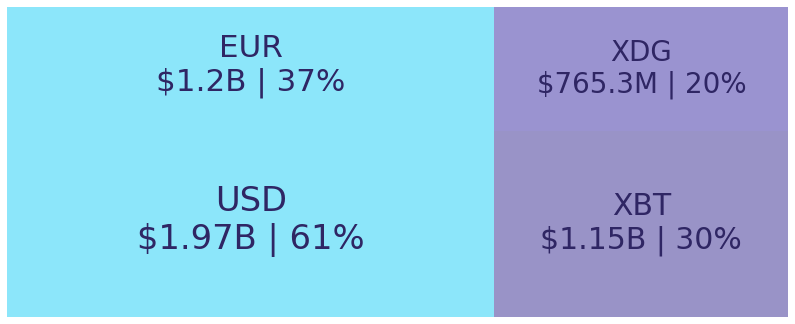Figure 2: Mid-size trading assets: (measured in USD) (April 20 2021)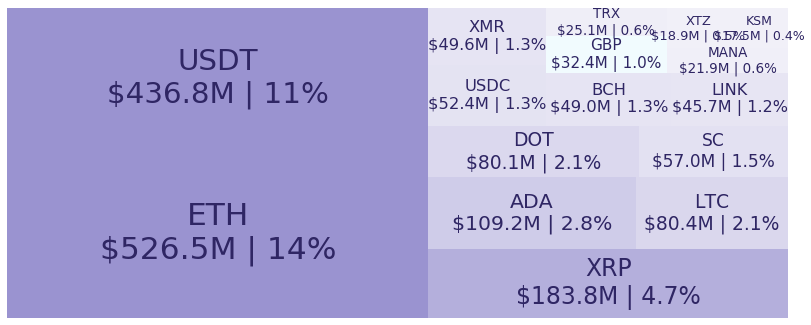Figure 3: Smallest trading assets: (measured in USD) (April 20 2021)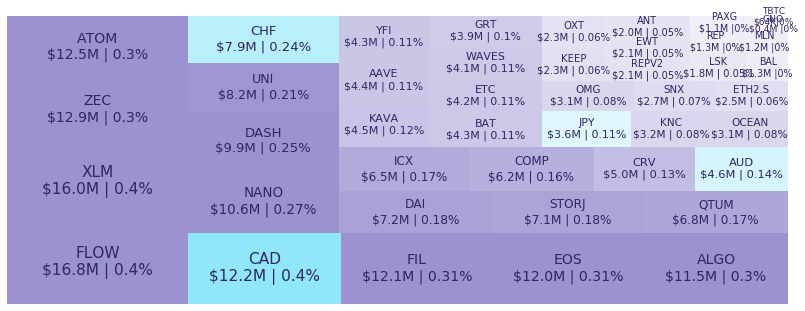Spread percentage is the width of the bid/ask spread divided by the bid/ask midpoint. The values are generated by taking the median spread percentage over each minute, then the average of the medians over the day.

Figure 4: Average spread % by pair (April 20 2021)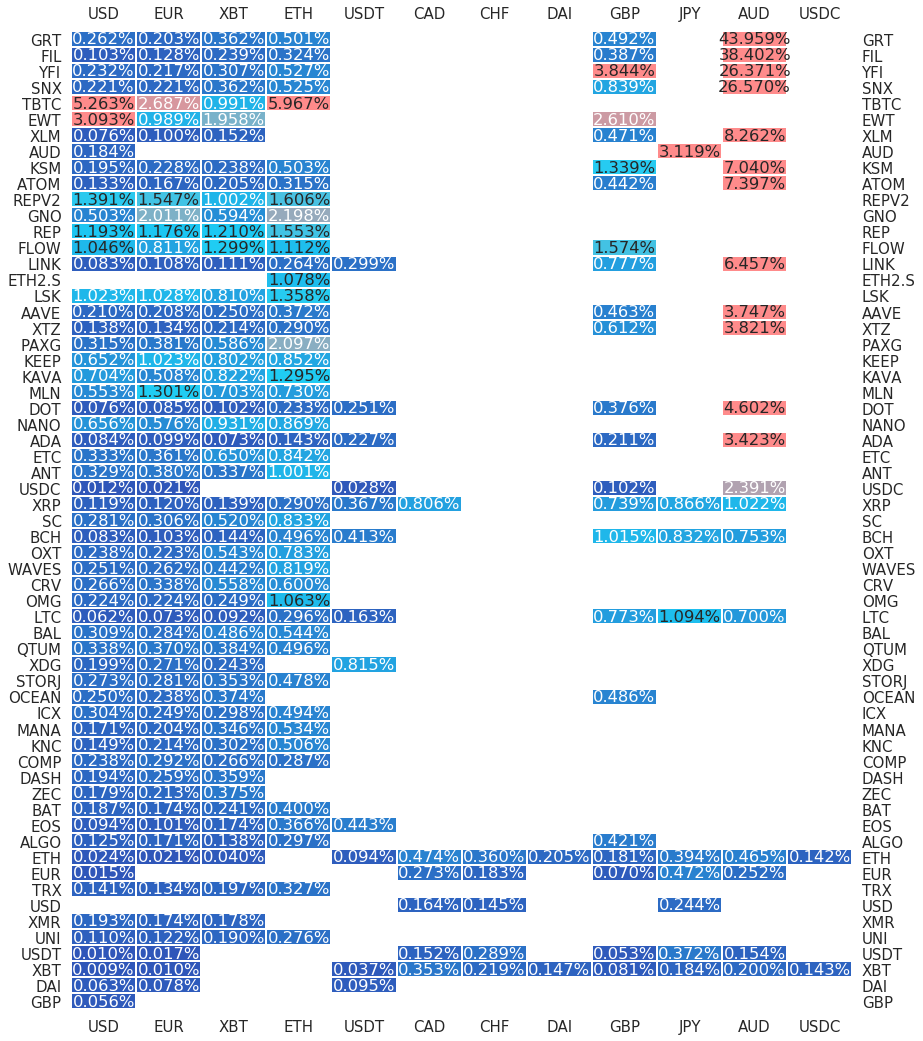.

#########. Returns and Volume ############################################

## Returns and Volume

Figure 5: Returns of the four highest volume pairs (April 20 2021)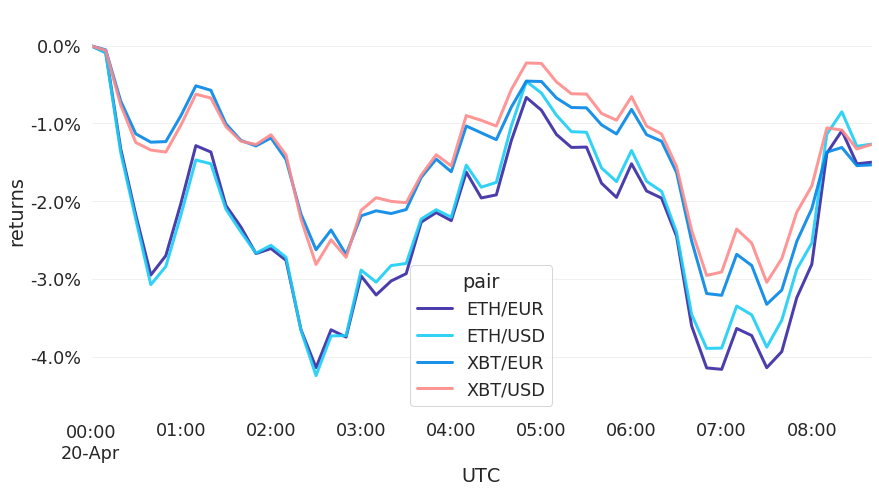Figure 6: Volume of the major currencies and an average line that fits the data to a sinusoidal curve to show the daily volume highs and lows (April 20 2021)